# PolynomialsPage 1

#### WATCH ALL SLIDES

Slide 1Polynomials

Defining Polynomials

Slide 2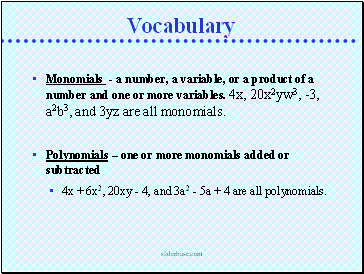Monomials - a number, a variable, or a product of a number and one or more variables. 4x, 20x2yw3, -3, a2b3, and 3yz are all monomials.

Polynomials – one or more monomials added or subtracted

4x + 6x2, 20xy - 4, and 3a2 - 5a + 4 are all polynomials.

Vocabulary

Slide 3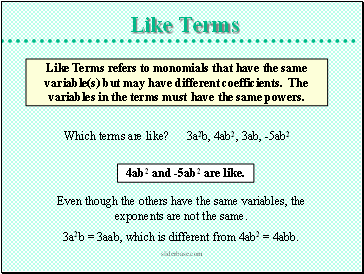## Like Terms

Like Terms refers to monomials that have the same variable(s) but may have different coefficients. The variables in the terms must have the same powers.

Which terms are like? 3a2b, 4ab2, 3ab, -5ab2

4ab2 and -5ab2 are like.

Even though the others have the same variables, the exponents are not the same.

3a2b = 3aab, which is different from 4ab2 = 4abb.

Slide 4Like Terms

Constants are like terms.

Which terms are like? 2x, -3, 5b, 0

-3 and 0 are like.

Which terms are like? 3x, 2x2, 4, x

3x and x are like.

Which terms are like? 2wx, w, 3x, 4xw

2wx and 4xw are like.

Slide 5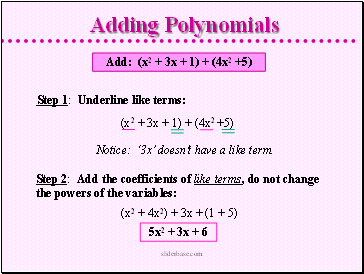Add: (x2 + 3x + 1) + (4x2 +5)

Step 1: Underline like terms:

Step 2: Add the coefficients of like terms, do not change the powers of the variables:

(x2 + 3x + 1) + (4x2 +5)

Notice: ‘3x’ doesn’t have a like term.

(x2 + 4x2) + 3x + (1 + 5)

5x2 + 3x + 6

Slide 6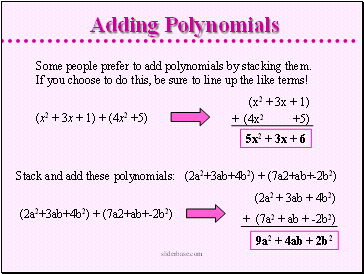Some people prefer to add polynomials by stacking them. If you choose to do this, be sure to line up the like terms!

(x2 + 3x + 1) + (4x2 +5)

5x2 + 3x + 6

(x2 + 3x + 1)

+ (4x2 +5)

Stack and add these polynomials: (2a2+3ab+4b2) + (7a2+ab+-2b2)

(2a2+3ab+4b2) + (7a2+ab+-2b2)

(2a2 + 3ab + 4b2)

+ (7a2 + ab + -2b2)

9a2 + 4ab + 2b2

Slide 7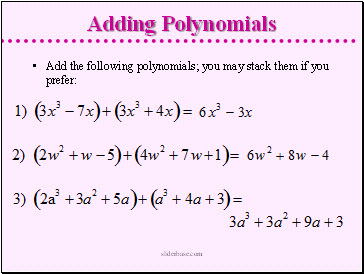Add the following polynomials; you may stack them if you prefer:

Slide 8## Subtracting Polynomials

Subtract: (3x2 + 2x + 7) - (x2 + x + 4)

Step 1: Change subtraction to addition (Keep-Change-Change.).

Step 2: Underline OR line up the like terms and add.

(3x2 + 2x + 7) + (- x2 + - x + - 4)

(3x2 + 2x + 7)

+ (- x2 + - x + - 4)

2x2 + x + 3

Slide 9Subtracting Polynomials

Go to page:
1  2